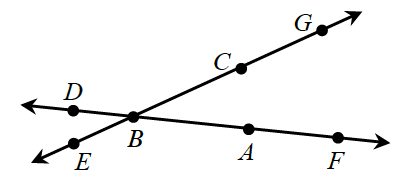### Home > INT2 > Chapter 1 > Lesson 1.1.2 > Problem1-16

1-16.

Multiple Choice: Read the Math Notes box in this lesson and then examine the diagram at right. Which angle below is another name for $∠ABC$?

1. $∠ABE$

2. $∠GBD$

3. $∠FBG$

4. $∠EBC$

5. none of theseTrace the points in order to see if they make the same angle as $∠ABC$.

C